# Conditional probability: why is it defined like that?

So, you want to calculate the probability of an event knowing that another has happened. There is a formula for that, it is called conditional probability, but why is it the way it is? Let’s first write down the definition of conditional probability: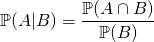We need to wonder: what does the happening of eventtell about the odds of happening of event? How much more likelybecomes ifhappens? Think in terms of howaffects.

Ifandare independent, then knowing something about B will not tell us anything at all about, at least not that we did not know already. In this case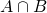is empty and thus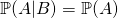. This makes sense! In fact, consider this example: how does me buying a copybook affects the likelihood that your grandma is going to buy a frying pan? It does not: the first event has no influence on the second, thus the conditional probability is just the same as the normal probability of the first event.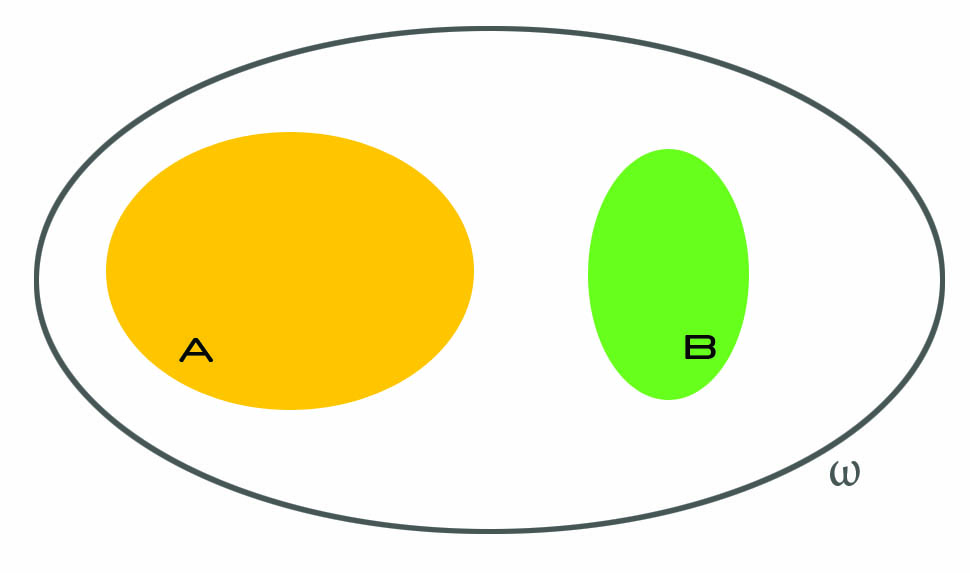Ifandare not independent, several things can happen, and that is where things get interesting. We know that B happened, and we should now think as ifwas our whole universe. The idea is: we already know what are the odds of, right? It is just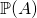. But how do they increase if we know that we do not really have to consider all possible events, but just a subset of them? As an example, think ofversusknowing that all balls are red. This makes a huge difference, right? (As an aside, that is what we mean when we say that probability is a measure of our ignorance.)

So anyway, now we ask: what is the probability of? Well, it would just be, but we must account for the fact that we now live inside, and everything that is outside it is as if it did not existed. Soactually becomes: we only care about the part ofthat is inside, because that is where we live now.

But, there is a caveat. We are thinking as ifwas the whole universe but, in terms of probabilities, it actually is not, because nobody has informed the probability distribution. In fact, we computeprecisely because we still live in the bigger universe, but we need to account for the fact thatis our real universe now. That is why we need a re-scaling factor: something that will scaleto make it numerically correct, to account for the fact thatis our current universe. This is what the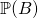at the denominator does.

In fact, the factor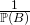accounts for how much relevant the information thathappened is. If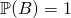, it means that, for probability purposes,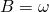– the switch of universe was just apparent! A further consequence is that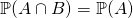, becauseis basically inside(apart from silly null-measure caveats). In turn, this has the consequence of making. This makes sense: ifis sure to happen, then what does it tell us about the odds of something else? As an example, if we are considering strings of digits (), what is the likelihood that a certain string is made of ones or twos, knowing that it is made out of digits ()? It sounds tautological, and it certainly is.

What about athat is big, yet? This is trickier, as it mostly depends on the interplay betweenand. But you are not on a university level website to read about inverse proportionality, are you?

Another case worth inspection is when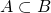. In that case,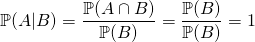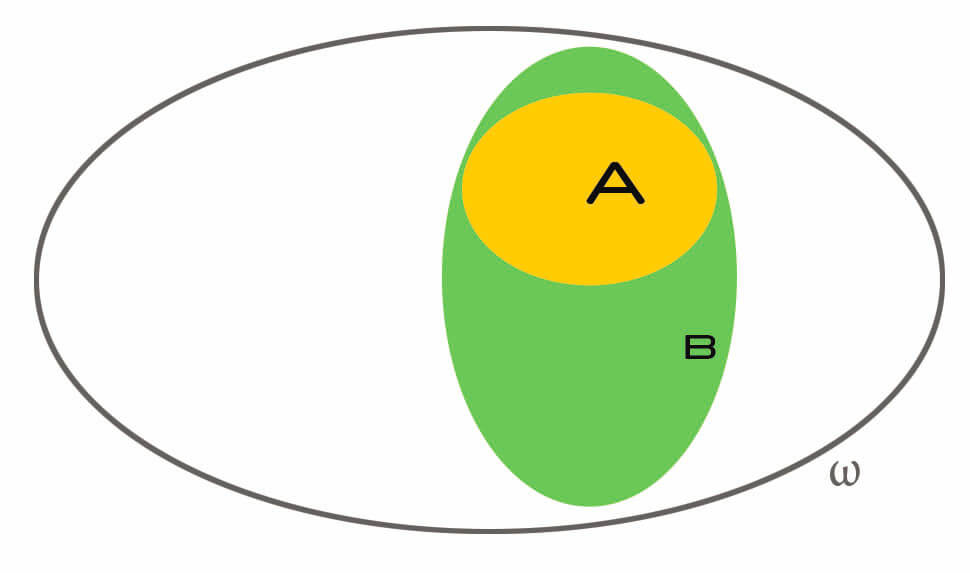Makes sense, right? Ifhappened, andis inside it, then clearlymust happen as well. If I bought a red umbrella, what are the odds that I bought a generic umbrella as a consequence? Full, yep.

Finally, let’s consider the case in whichis very small. Suppose that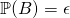and. Anat the denominator will make the resulting fraction become significantly bigger.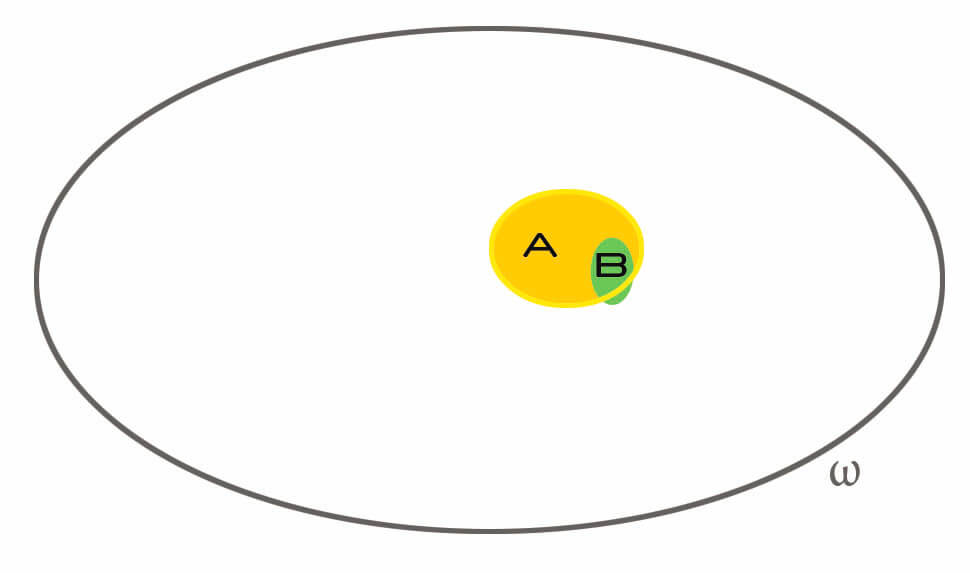The idea here is that ifis very narrow, if it talks about something very unlikely, and it happened, this greatly influences the overall conditional probability. What are the odds that I get hospitalized in Japan as a 25 years old man? Very low. What are the odds that today there is an earthquake in Japan? Very low. What are the odds that I get hospitalized in Japan, knowing that today an earthquake happened? Quite high. That’s the idea: the moreis unlikely, the higher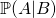tends to be. In a sense, the more narrowis, the higher the amount of information it brings knowing that it happened.